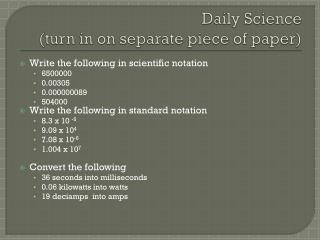DownloadDownload PresentationDaily Science (turn in on separate piece of paper)

# Daily Science (turn in on separate piece of paper)

Télécharger la présentation## Daily Science (turn in on separate piece of paper)

- - - - - - - - - - - - - - - - - - - - - - - - - - - E N D - - - - - - - - - - - - - - - - - - - - - - - - - - -
##### Presentation Transcript

1. Daily Science(turn in on separate piece of paper) • Write the following in scientific notation • 6500000 • 0.00305 • 0.000000089 • 504000 • Write the following in standard notation • 8.3 x 10 -5 • 9.09 x 104 • 7.08 x 10-6 • 1.004 x 107 • Convert the following • 36 seconds into milliseconds • 0.06 kilowatts into watts • 19 deciamps into amps

2. Motion Pg. 1

3. Frame of reference Used to describe motion in reference to something. A fixed point

4. Distance vs. Displacement Distance is the total path taken by an object Displacement measures the distance between starting and ending points

5. Speed vs. Velocity Speed describes how fast an object moves (called a scalar quantity) Velocity describes speed and direction (called a vector quantity)

6. Speed and velocity Formula: distance/time V=d/t Units? ____

7. Velocity Practice Find the velocity in meters per second of a swimmer who swims 110 m toward the shore in 72 seconds. Find the velocity in meters per second of a baseball thrown 38 m from third base to first base in 1.7 sec. Calculate the distance in meters that a cyclist would travel in 5.00 hours at an average velocity of 12.0 km/h to the southwest.

8. Daily Science pg. 4 A car is traveling east for a distance of 890 meters. It will take the car 37 seconds to travel the distance. What is the cars velocity? A line of dominos is set up on a table. You push the first domino to make the rest fall. The dominos fall at a velocity of 0.2 m/s down the table. It takes 15 seconds for the last domino to fall. How long was the domino line? If you run with an average velocity of 2 m/s toward a goal, a total distance of 400 m, how long would it take you?

9. Resultant velocitypg.1 • Velocities can be combined to describe the resultant velocity. • Ex. You are riding a bus that is traveling 15 m/s east. You stand up and walk toward the back of the bus (now walking east) at 1 m/s. Are you still traveling at the same speed? • To find the resulting velocity, just add the velocities together.

10. Instantaneous Speed The speed at a given point. Car speedometers measure the speed at a given point.

11. Distance vs. Time graph X- axis: Y- axis: Type of graph:

12. Practice Data for a runner: When is the runner going the fastest? When is the runner moving slower? When is the runner standing still?

13. Slope The slope of a distance vs. time graph equals speed. A slope can be determined by taking the change in the y variable and dividing it by the change in the x variable. Slope = ∆y/ ∆ x (rise/run)

14. Slope practice What is the slope of the line? What is the speed of the bug between 2 and 4 seconds? What is the speed at 8 seconds? What might the speed be at 11 seconds?

15. Slope